Function Repository Resource:

# GeodesicSphereGraph

Create a graph of an order-n geodesic sphere

Contributed by: Jan Mangaldan
 ResourceFunction["GeodesicSphereGraph"][n] gives a graph corresponding to an order-n geodesic sphere. ResourceFunction["GeodesicSphereGraph"][base,n] gives a graph corresponding to an order-n geodesic sphere based on the polyhedron base.

## Details and Options

GeodesicSphereGraph[base, n] generates a three-dimensional graph corresponding to a geodesic sphere, also called a geodesic dome.
"Icosahedron" is the default value for base.
Possible values of base are "Tetrahedron", "Octahedron" or "Icosahedron".
GeodesicSphereGraph takes the same options as Graph3D.
With the setting VertexCoordinates"Embedded", coordinates corresponding to the vertices of the geodesic sphere are generated with a special method.

## Examples

### Basic Examples (3)

Generate an order-2 geodesic sphere:

 In:=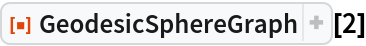Out=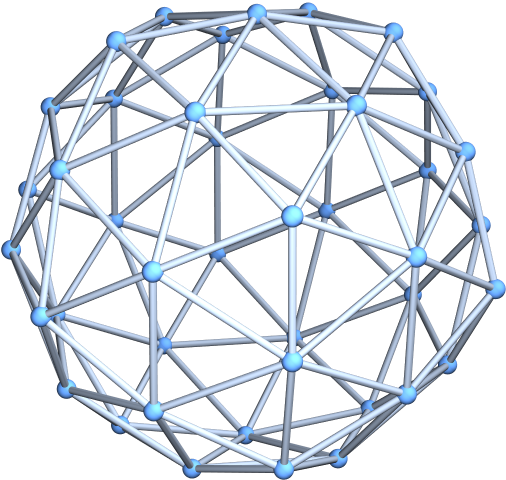Generate an order-2 geodesic sphere with specially computed coordinates:

 In:=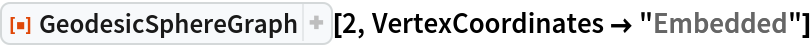Out=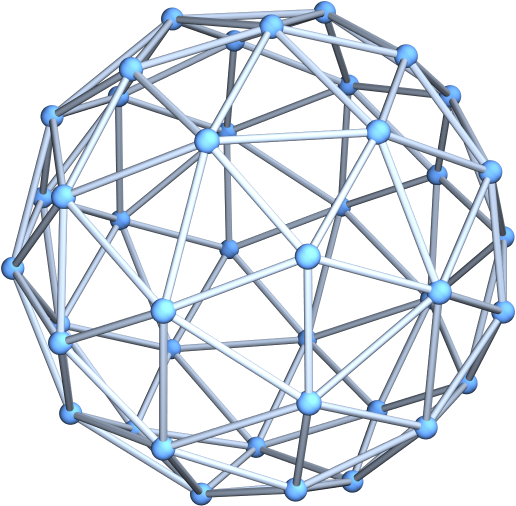Generate an order-3 geodesic sphere with an octahedral base:

 In:=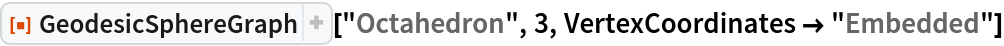Out=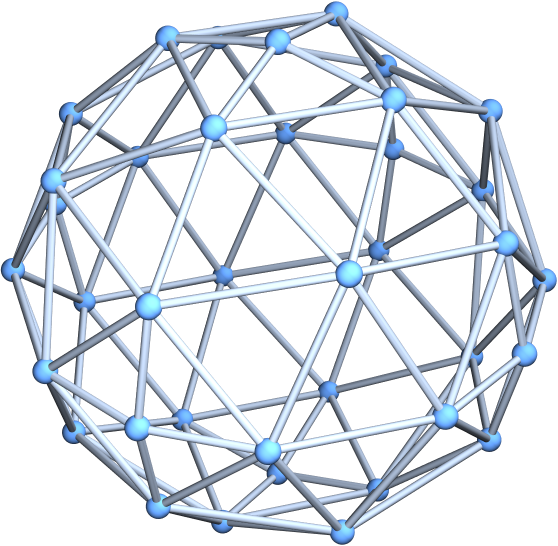### Scope (2)

Generate an icosahedral graph:

 In:=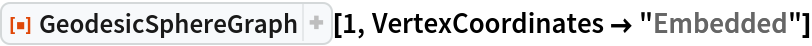Out=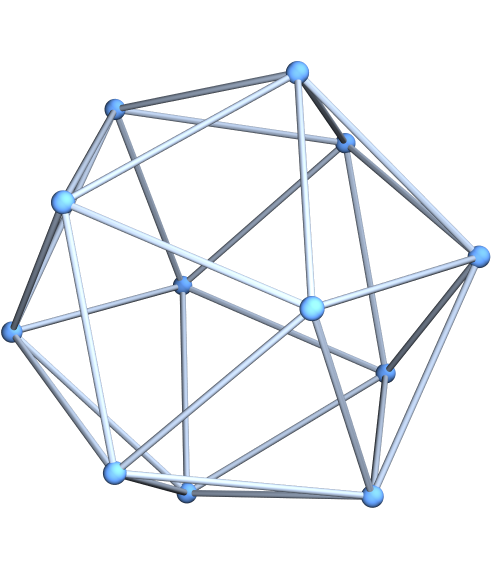Convert to a Graph object:

 In:=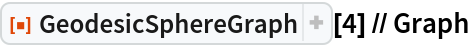Out=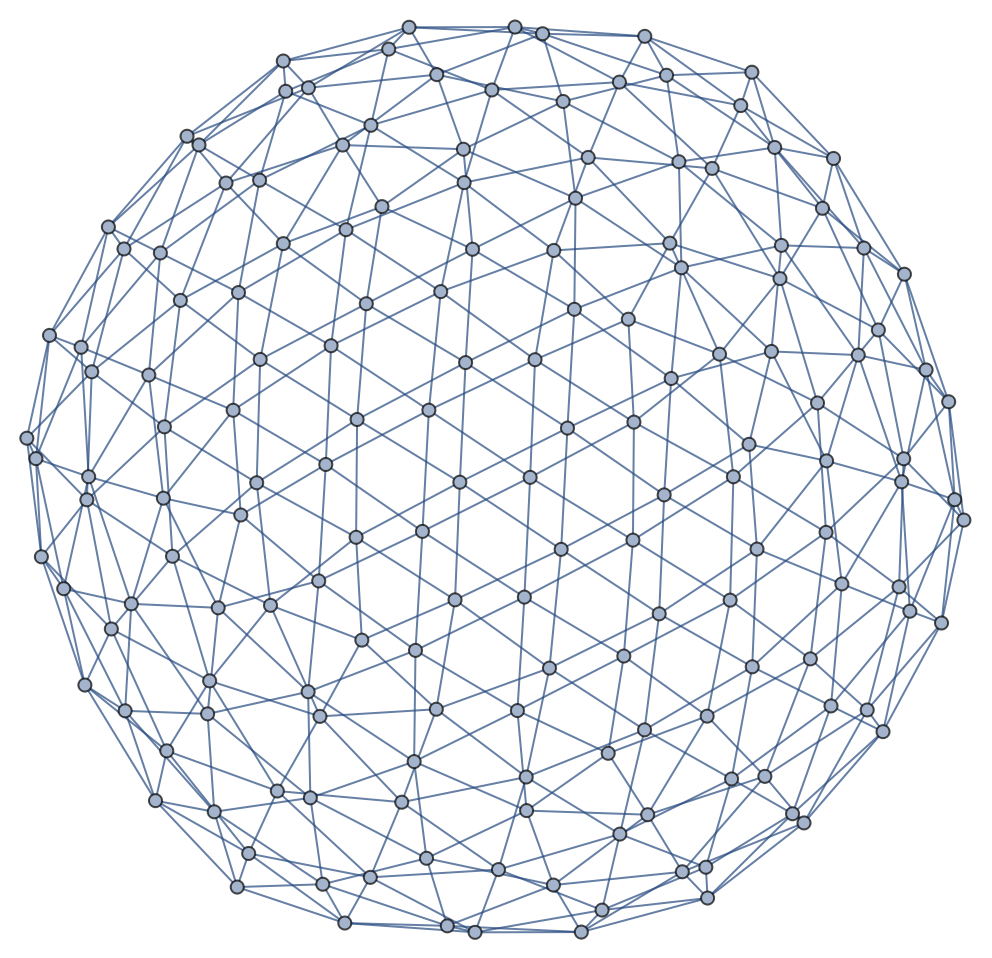### Options (4)

#### GraphLayout (1)

Specify various layouts for the geodesic sphere graph:

 In:=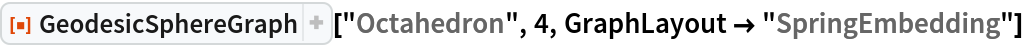Out=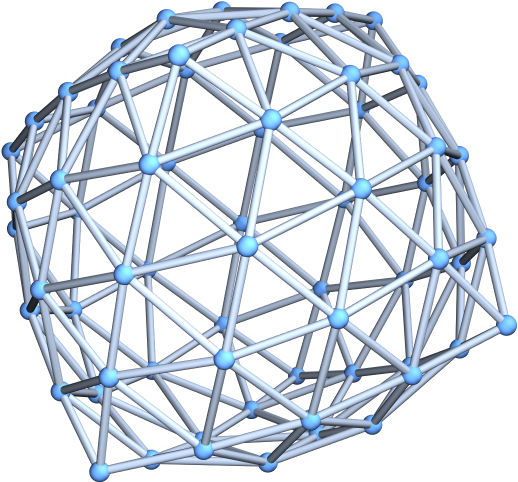In:=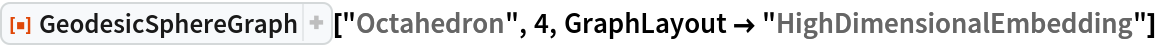Out=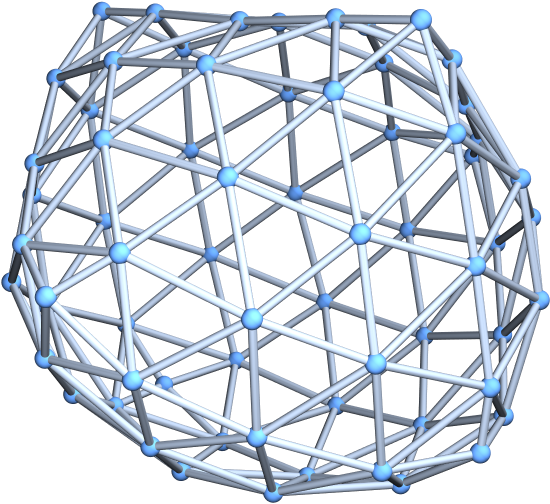#### PlotTheme (1)

Use a large graph theme:

 In:=Out=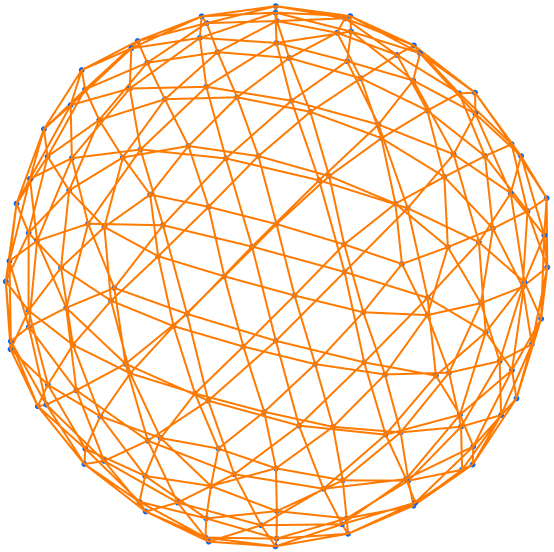#### VertexCoordinates (2)

By default, vertex coordinates are computed automatically, depending on the setting for GraphLayout:

 In:=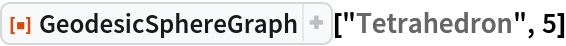Out=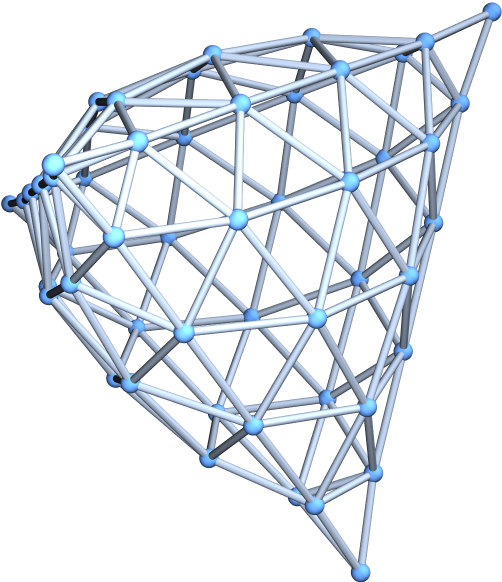Use specially computed coordinates for the vertices:

 In:=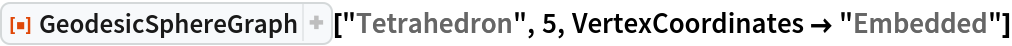Out=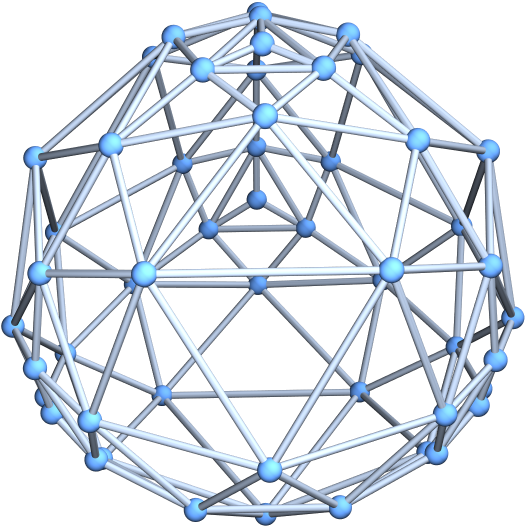## Version History

• 1.0.0 – 02 March 2020